# 买空调千万别被一级能效忽悠了，这样算才知道省不省钱！• 1匹一级耗电=(2300/3.6)=639W，二级耗电=(2300/3.4)=677W;三级耗电=(2300/3.2)=719W。
• 正1匹一级耗电=(2500/3.6)=695W，二级耗电=(2500/3.4)=735W;三级耗电=(2500/3.2)=781W。
• 大1匹一级耗电=(2600/3.6)=722W，二级耗电=(2600/3.4)=765W;三级耗电=(2600/3.2)=813W。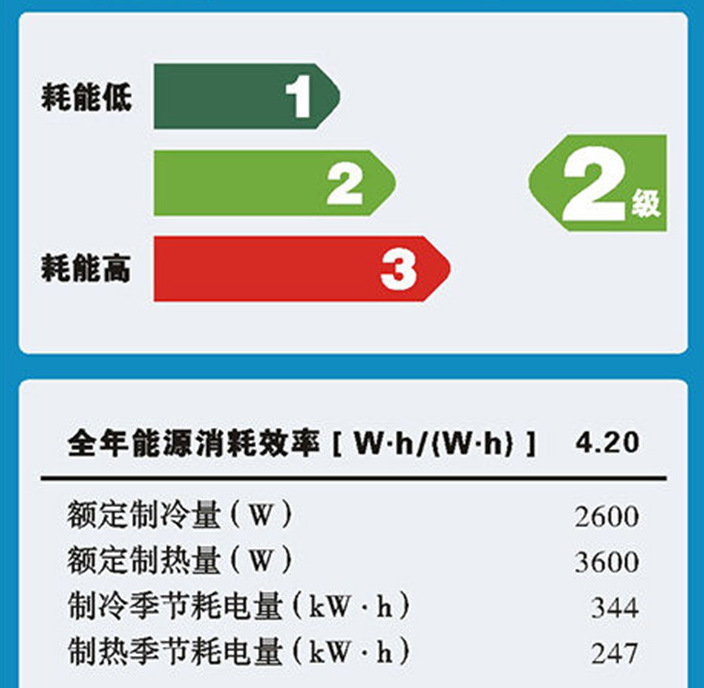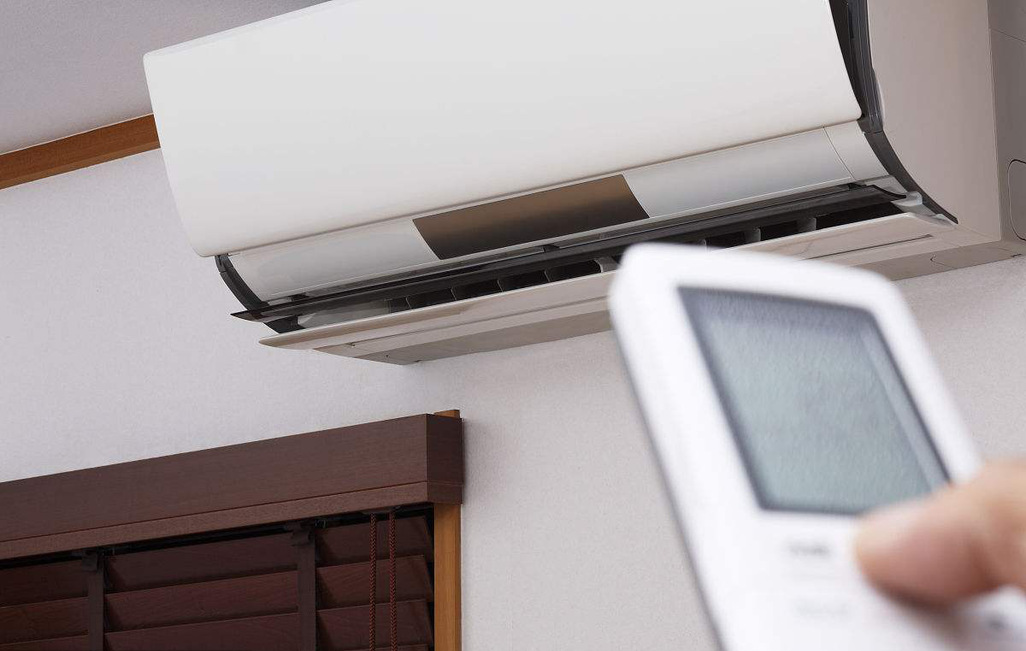### 1、美的i青春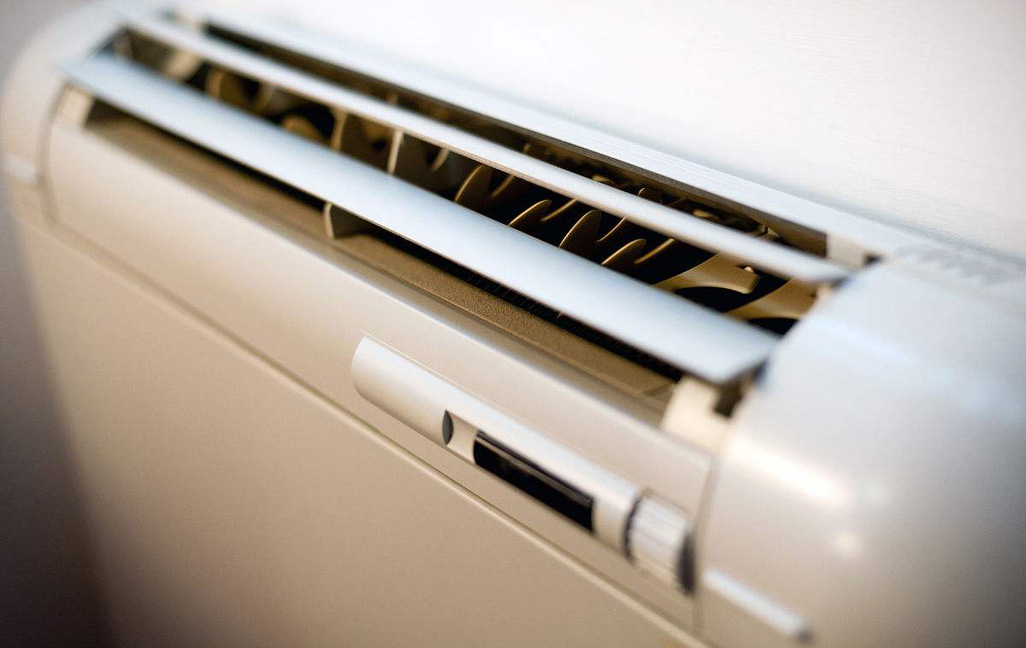### 3、科龙经典一级能效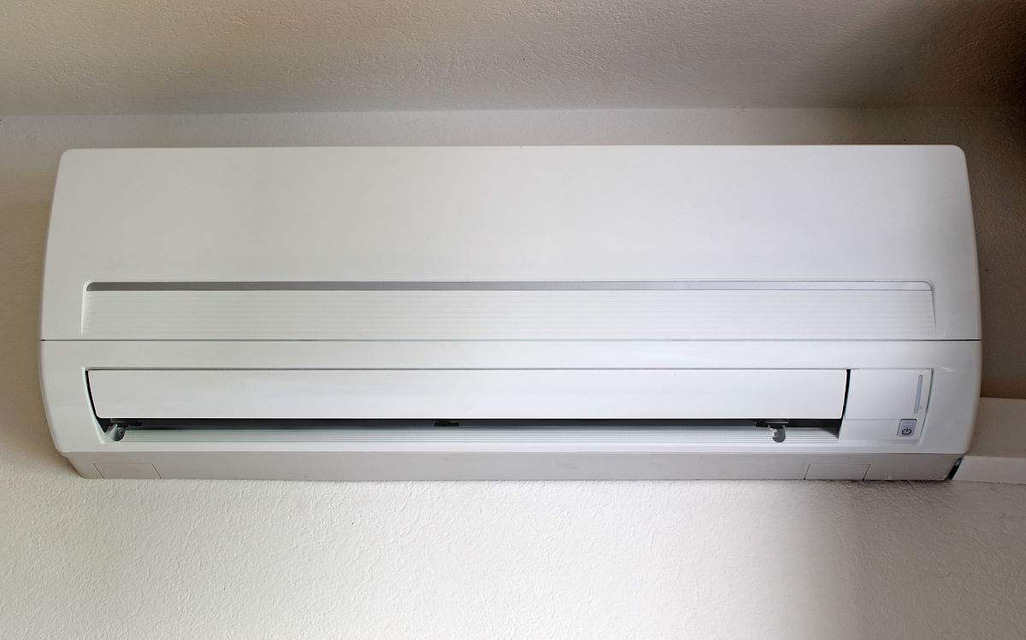#### 4、奥克斯经典1级能效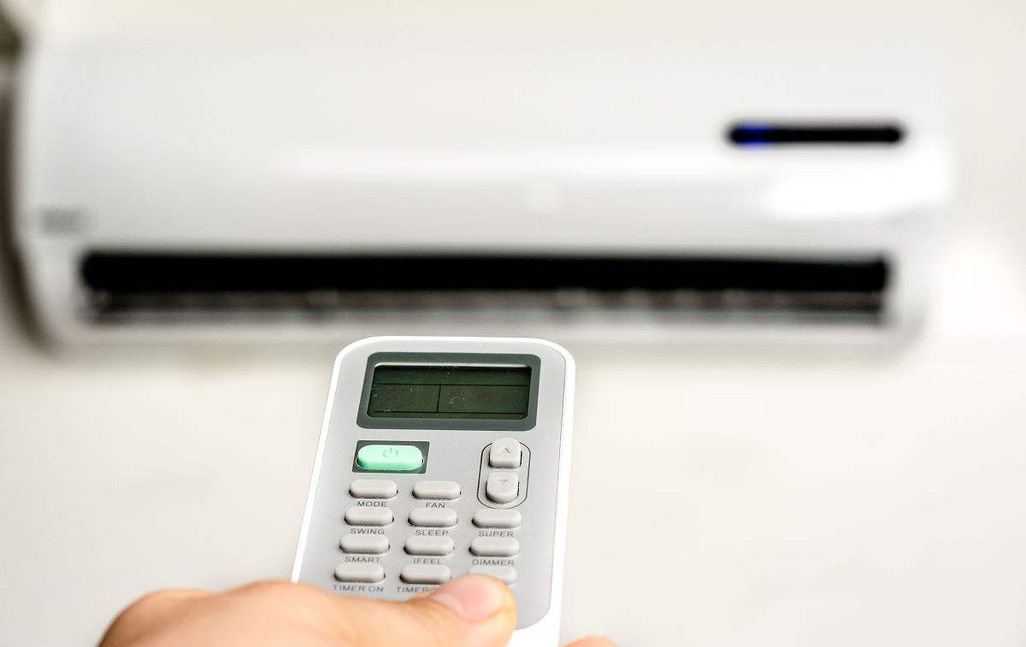### 5、海尔智能空调

6、小米互联网智能空调

#### 打开微信扫一扫